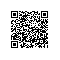# 手把手实战：利用LM神经网络算法自动识别窃电用户（附代码）

## 背景与挖掘目标

### 背景

1. 传统的防窃漏电方法主要通过定期巡检、定期校验电表、用户举报窃电等方法来发现窃电或计量装置故障。

2. 但这种方法对人的依赖性太强，抓窃查漏的目标不明确。

3. 通过采集电量异常、负荷异常、终端报警、主站报警、线损异常等信息，建立数据分析模型，来实时监测窃漏电情况和发现计量装置的故障。

### 目标

1. 归纳出窃漏电用户的关键特征，构建窃漏电用户的识别模型。

2. 利用实时检测数据，调用窃漏电用户识别模型实现实时诊断。

## 分析方法与过程

### 分析方法

1. 窃漏电用户在电力计量自动化系统的监控大用户中只占一小部分，同时某些大用户也不可能存在窃漏电行为，如银行、税务、学校和工商等非居民类别，故在数据预处理时有必要将这些类别用户剔除。

2. 系统中的用电负荷不能直接体现出用户的窃漏电行为，终端报警存在很多误报和漏报的情况，故需要进行数据探索和预处理，总结窃漏电用户的行为规律，再从数据中提炼出描述窃漏电用户的特征指标。

3. 最后结合历史窃漏电用户信息，整理出识别模型的专家样本数据集，再进一步构建分类模型，实现窃漏电用户的自动识别。窃漏电用户识别流程如图6．1所示，主要包話以下步骤。

### 过程整理

1. 从电力计量自动化系统、营销系统有选择性地抽取部分大用户用电负荷、终端报警及违约窃电处罚信息等原始数据。

2. 对样本数据探索分析，剔除不可能存在窃漏电行为行业的用户，即白名单用户，初步审视正常用户和窃漏电用户的用电特征。

3. 对样本数据进行预处理，包括数据清洗、缺失值处理和数据变换。

4. 构建专家样本集。

5. 构建窃漏电用户识别模型。

6. 在线监测用户用电负荷及终端报警，调用模型实现实时诊断。

### 数据探索分析

#### 2. 周期性分析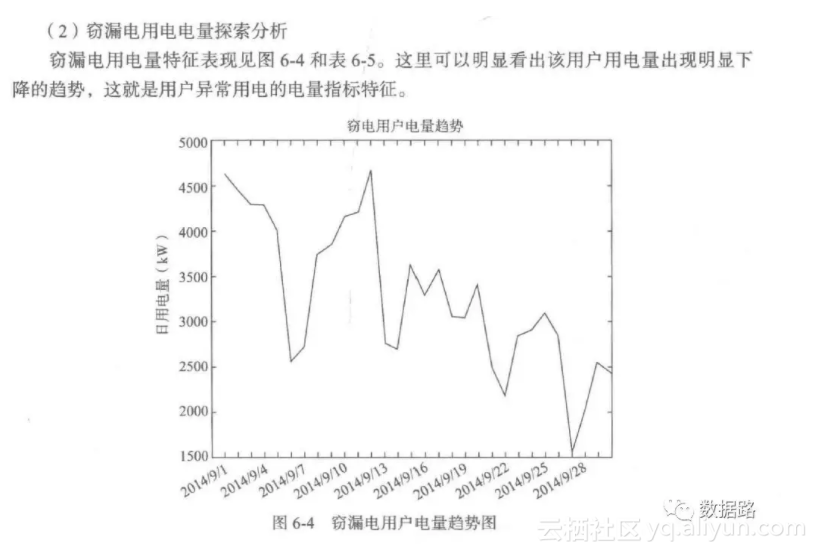### 数据预处理

#### 1. 数据清洗

• 非居民不存在透漏电，如学校，邮局等等
• 结合业务，节假日会比平时偏低，为了达到更好效果，去处掉节假日。

#### 2. 缺失值处理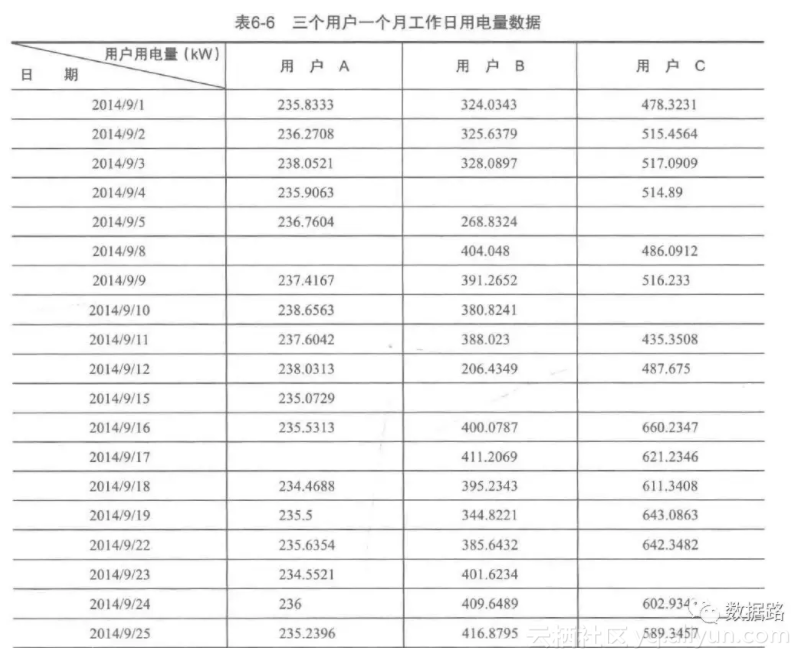• 首先，确定原始数据集中的自变量和因变量，
• 取出缺失值前后五个数据（空值和不存在，去掉）
• 取出十个数据为一组，采用拉格朗日多项式差值公式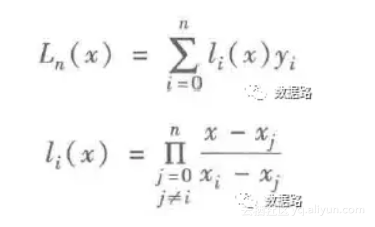#-*- coding: utf-8 -*-
#拉格朗日插值代码
import pandas as pd #导入数据分析库Pandas
from scipy.interpolate import lagrange #导入拉格朗日插值函数

inputfile = '/home/kesci/input/date14037/missing_data.xls' #输入数据路径,需要使用Excel格式；
outputfile = '/home/kesci/work/missing_data_processed.xls' #输出数据路径,需要使用Excel格式,这里在科赛上，所以本地运行需要修改路径

print(data)
#自定义列向量插值函数
#s为列向量，n为被插值的位置，k为取前后的数据个数，默认为5
def ployinterp_column(s, n, k=5):
y = s[list(range(n-k, n)) + list(range(n+1, n+1+k))] #取数，注意这类（）取最左，不取最右。
y = y[y.notnull()] #剔除空值
return lagrange(y.index, list(y))(n) #插值并返回插值结果

#逐个元素判断是否需要插值
for i in data.columns:
for j in range(len(data)):
if (data[i].isnull())[j]: #如果为空即插值。
data[i][j] = ployinterp_column(data[i], j)

print(data)
0         1         2
0   235.8333  324.0343  478.3231
1   236.2708  325.6379  515.4564
2   238.0521  328.0897  517.0909
3   235.9063       NaN  514.8900
4   236.7604  268.8324       NaN
5        NaN  404.0480  486.0912
6   237.4167  391.2652  516.2330
7   238.6563  380.8241       NaN
8   237.6042  388.0230  435.3508

———————————数据处理前后————————————
0           1           2
0   235.833300  324.034300  478.323100
1   236.270800  325.637900  515.456400
2   238.052100  328.089700  517.090900
3   235.906300  203.462116  514.890000
4   236.760400  268.832400  493.352591
5   237.151181  404.048000  486.091200
6   237.416700  391.265200  516.233000
7   238.656300  380.824100  493.342382
8   237.604200  388.023000  435.350800

• 数据变换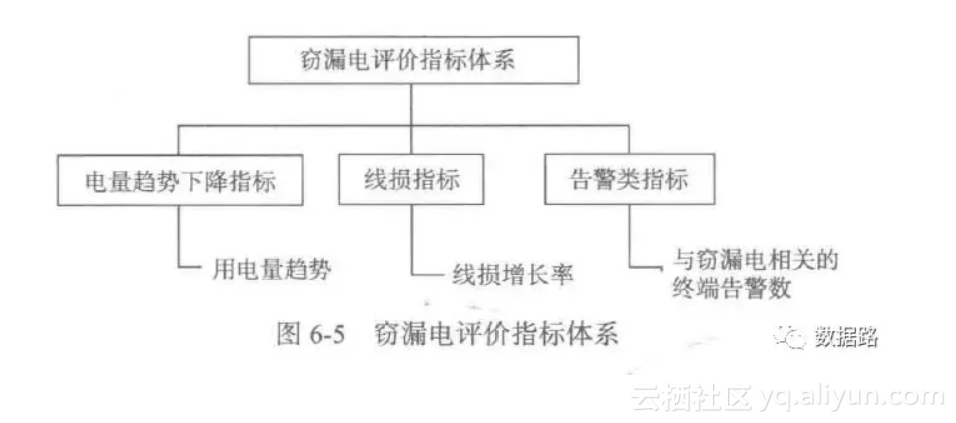• 用电量趋势下降指标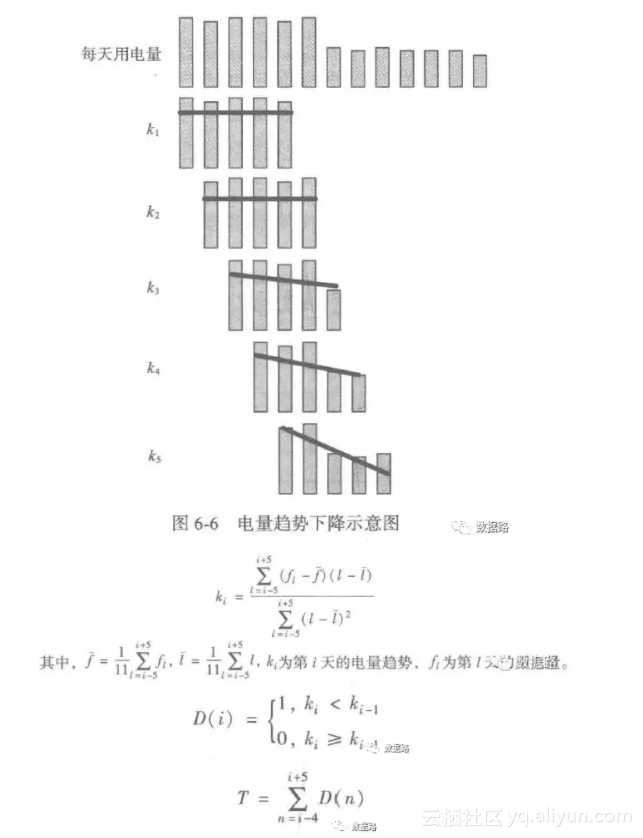#-*- coding: utf-8 -*-

#拉格朗日插值代码

import pandas as pd #导入数据分析库Pandas

from scipy.interpolate import lagrange #导入拉格朗日插值函数

inputfile = '/home/kesci/input/date14037/missing_data.xls' #输入数据路径,需要使用Excel格式；

outputfile = '/home/kesci/work/missing_data_processed.xls' #输出数据路径,需要使用Excel格式,这里在科赛上，所以本地运行需要修改路径

print(data)

#自定义列向量插值函数

#s为列向量，n为被插值的位置，k为取前后的数据个数，默认为5

def ployinterp_column(s, n, k=5):

y = s[list(range(n-k, n)) + list(range(n+1, n+1+k))] #取数，注意这类（）取最左，不取最右。

y = y[y.notnull()] #剔除空值

return lagrange(y.index, list(y))(n) #插值并返回插值结果

#逐个元素判断是否需要插值

for i in data.columns:

for j in range(len(data)):

if (data[i].isnull())[j]: #如果为空即插值。

data[i][j] = ployinterp_column(data[i], j)

print(data)

0         1         2

0   235.8333  324.0343  478.3231

1   236.2708  325.6379  515.4564

2   238.0521  328.0897  517.0909

3   235.9063       NaN  514.8900

4   236.7604  268.8324       NaN

5        NaN  404.0480  486.0912

6   237.4167  391.2652  516.2330

7   238.6563  380.8241       NaN

8   237.6042  388.0230  435.3508

———————————数据处理前后————————————

0           1           2

0   235.833300  324.034300  478.323100

1   236.270800  325.637900  515.456400

2   238.052100  328.089700  517.090900

3   235.906300  203.462116  514.890000

4   236.760400  268.832400  493.352591

5   237.151181  404.048000  486.091200

6   237.416700  391.265200  516.233000

7   238.656300  380.824100  493.342382

8   237.604200  388.023000  435.350800
• ##### 线损指标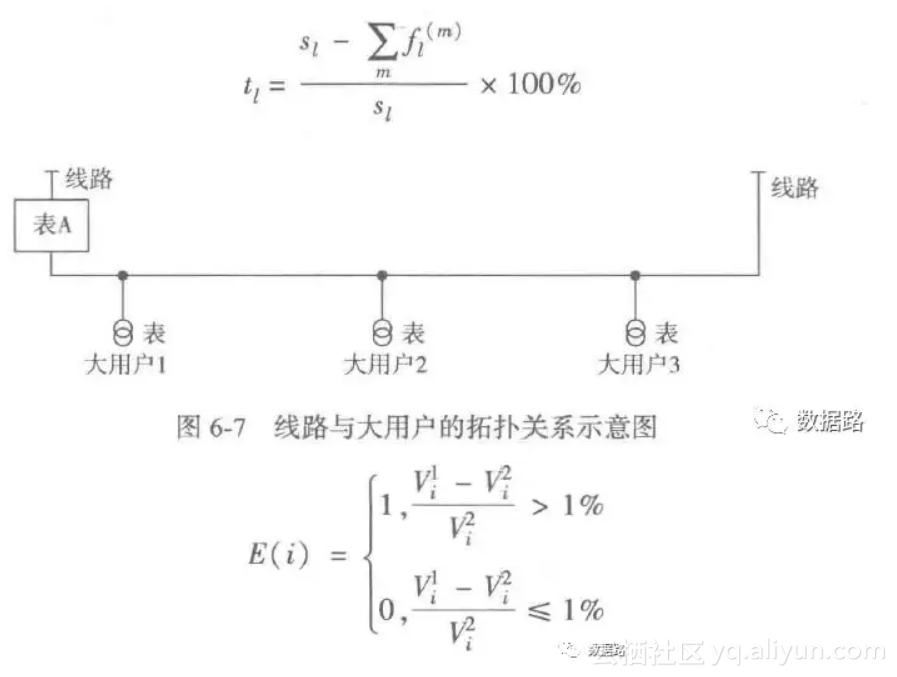#### 1. 构建窃漏电用户识别模型

• 数据划分

• LM神经网络

#-*- coding: utf-8 -*-
import matplotlib.pyplot as plt
import pandas as pd
from random import shuffle

def cm_plot(y, yp):

from sklearn.metrics import confusion_matrix #导入混淆矩阵函数

cm = confusion_matrix(y, yp) #混淆矩阵

import matplotlib.pyplot as plt #导入作图库
plt.matshow(cm, cmap=plt.cm.Greens) #画混淆矩阵图，配色风格使用cm.Greens，更多风格请参考官网。
plt.colorbar() #颜色标签

for x in range(len(cm)): #数据标签
for y in range(len(cm)):
plt.annotate(cm[x,y], xy=(x, y), horizontalalignment='center', verticalalignment='center')

plt.ylabel('True label') #坐标轴标签
plt.xlabel('Predicted label') #坐标轴标签
return plt

datafile = '/home/kesci/input/date14037/model.xls'
data = data.as_matrix()
shuffle(data)

p = 0.8 #设置训练数据比例
train = data[:int(len(data)*p),:]#多维数据的切片方法
test = data[int(len(data)*p):,:]#逗号左边，代表行，右边代表列

#构建LM神经网络模型
from keras.models import Sequential #导入神经网络初始化函数
from keras.layers.core import Dense, Activation #导入神经网络层函数、激活函数

netfile = '/home/kesci/input/date14037/net.model' #构建的神经网络模型存储路径

net = Sequential() #建立神经网络
net.add(Dense(input_dim = 3, output_dim = 10)) #添加输入层（3节点）到隐藏层（10节点）的连接
net.add(Dense(input_dim = 10, output_dim = 1)) #添加隐藏层（10节点）到输出层（1节点）的连接

net.fit(train[:,:3], train[:,3], nb_epoch=100, batch_size=1) #训练模型，循环1000次，不用于书籍源代码，这里需要删除class这个值才能正常运行
net.save_weights(netfile) #保存模型

predict_result = net.predict_classes(train[:,:3]).reshape(len(train)) #预测结果变形
'''这里要提醒的是，keras用predict给出预测概率，predict_classes才是给出预测类别，而且两者的预测结果都是n x 1维数组，而不是通常的 1 x n'''

#导入自行编写的混淆矩阵可视化函数，具体见最上代码 cm_plot(y, yp)
def cm_plot(y, yp):

from sklearn.metrics import confusion_matrix #导入混淆矩阵函数

cm = confusion_matrix(y, yp) #混淆矩阵

import matplotlib.pyplot as plt #导入作图库
plt.matshow(cm, cmap=plt.cm.Greens) #画混淆矩阵图，配色风格使用cm.Greens，更多风格请参考官网。
plt.colorbar() #颜色标签

for x in range(len(cm)): #数据标签
for y in range(len(cm)):
plt.annotate(cm[x,y], xy=(x, y), horizontalalignment='center', verticalalignment='center')

plt.ylabel('True label') #坐标轴标签
plt.xlabel('Predicted label') #坐标轴标签
return plt

cm_plot(train[:,3], predict_result).show() #显示混淆矩阵可视化结果

from sklearn.metrics import roc_curve #导入ROC曲线函数

predict_result = net.predict(test[:,:3]).reshape(len(test))
fpr, tpr, thresholds = roc_curve(test[:,3], predict_result, pos_label=1)
plt.plot(fpr, tpr, linewidth=2, label = 'ROC of LM') #作出ROC曲线
plt.xlabel('False Positive Rate') #坐标轴标签
plt.ylabel('True Positive Rate') #坐标轴标签
plt.ylim(0,1.05) #边界范围
plt.xlim(0,1.05) #边界范围
plt.legend(loc=4) #图例
plt.show() #显示作图结果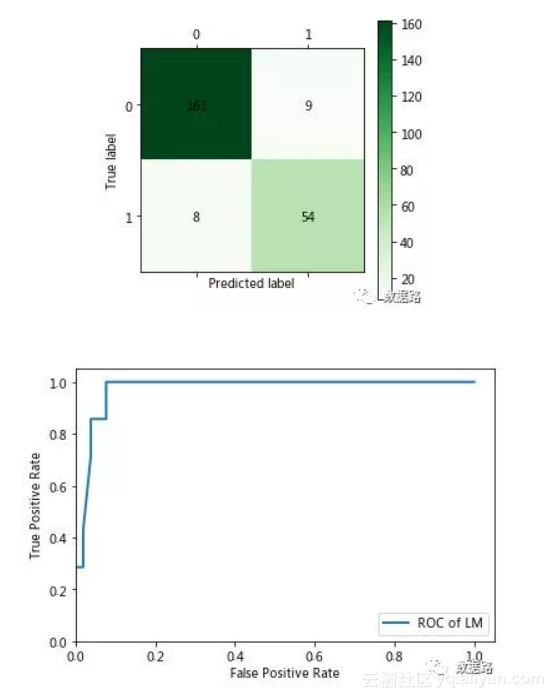##### CART决策树算法

#-*- coding: utf-8 -*-

import matplotlib.pyplot as plt

import pandas as pd

from random import shuffle

def cm_plot(y, yp):

from sklearn.metrics import confusion_matrix #导入混淆矩阵函数

cm = confusion_matrix(y, yp) #混淆矩阵

import matplotlib.pyplot as plt #导入作图库

plt.matshow(cm, cmap=plt.cm.Greens) #画混淆矩阵图，配色风格使用cm.Greens，更多风格请参考官网。

plt.colorbar() #颜色标签

for x in range(len(cm)): #数据标签

for y in range(len(cm)):

plt.annotate(cm[x,y], xy=(x, y), horizontalalignment='center', verticalalignment='center')

plt.ylabel('True label') #坐标轴标签

plt.xlabel('Predicted label') #坐标轴标签

return plt

datafile = '/home/kesci/input/date14037/model.xls'

data = data.as_matrix()

shuffle(data)

p = 0.8 #设置训练数据比例

train = data[:int(len(data)*p),:]#多维数据的切片方法

test = data[int(len(data)*p):,:]#逗号左边，代表行，右边代表列

#构建LM神经网络模型

from keras.models import Sequential #导入神经网络初始化函数

from keras.layers.core import Dense, Activation #导入神经网络层函数、激活函数

netfile = '/home/kesci/input/date14037/net.model' #构建的神经网络模型存储路径

net = Sequential() #建立神经网络

net.add(Dense(input_dim = 3, output_dim = 10)) #添加输入层（3节点）到隐藏层（10节点）的连接

net.add(Dense(input_dim = 10, output_dim = 1)) #添加隐藏层（10节点）到输出层（1节点）的连接

net.fit(train[:,:3], train[:,3], nb_epoch=100, batch_size=1) #训练模型，循环1000次，不用于书籍源代码，这里需要删除class这个值才能正常运行

net.save_weights(netfile) #保存模型

predict_result = net.predict_classes(train[:,:3]).reshape(len(train)) #预测结果变形

'''这里要提醒的是，keras用predict给出预测概率，predict_classes才是给出预测类别，而且两者的预测结果都是n x 1维数组，而不是通常的 1 x n'''

#导入自行编写的混淆矩阵可视化函数，具体见最上代码 cm_plot(y, yp)

def cm_plot(y, yp):

from sklearn.metrics import confusion_matrix #导入混淆矩阵函数

cm = confusion_matrix(y, yp) #混淆矩阵

import matplotlib.pyplot as plt #导入作图库

plt.matshow(cm, cmap=plt.cm.Greens) #画混淆矩阵图，配色风格使用cm.Greens，更多风格请参考官网。

plt.colorbar() #颜色标签

for x in range(len(cm)): #数据标签

for y in range(len(cm)):

plt.annotate(cm[x,y], xy=(x, y), horizontalalignment='center', verticalalignment='center')

plt.ylabel('True label') #坐标轴标签

plt.xlabel('Predicted label') #坐标轴标签

return plt

cm_plot(train[:,3], predict_result).show() #显示混淆矩阵可视化结果

from sklearn.metrics import roc_curve #导入ROC曲线函数

predict_result = net.predict(test[:,:3]).reshape(len(test))

fpr, tpr, thresholds = roc_curve(test[:,3], predict_result, pos_label=1)

plt.plot(fpr, tpr, linewidth=2, label = 'ROC of LM') #作出ROC曲线

plt.xlabel('False Positive Rate') #坐标轴标签

plt.ylabel('True Positive Rate') #坐标轴标签

plt.ylim(0,1.05) #边界范围

plt.xlim(0,1.05) #边界范围

plt.legend(loc=4) #图例

plt.show() #显示作图结果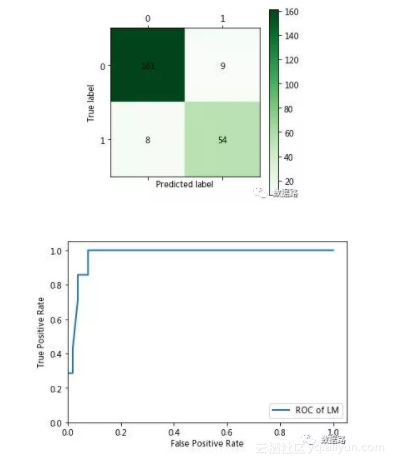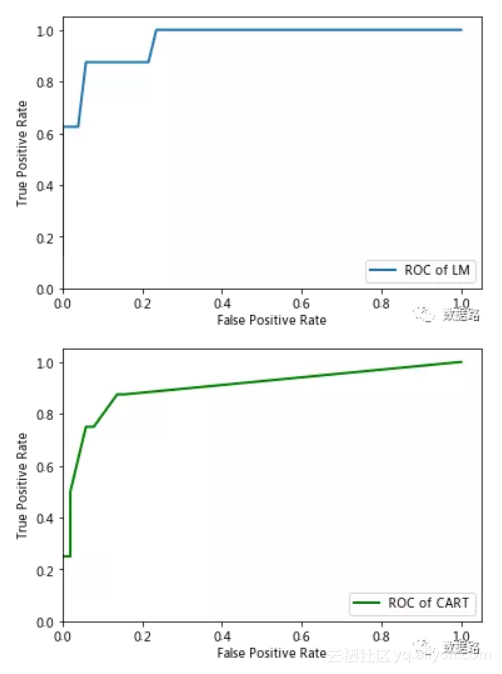1. 了解了数据挖掘算法中LM神经网络和CART决策树算法的实际应用场景

2. 但是还未深刻理解，这个两个算法背后原理，往后在学习《数据挖掘导论》时要理解。

3. 了解了识别模型优劣中的ROC比较方法，但是应该还会有更好的方式。

4. 这个案例，可以类推到汽车相关的偷漏税项目上。但是，自己实战时发现，目标原始数据很难去发现有效指标与建立评价指标，业务的理解转换能力不足

5. 目前，还在同步学习秦路的《七周数据分析师》希望能获得一些业务能力，帮助项目进行。